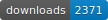# rtip[] (http://cran.r-project.org/package=rtip)

rtip is an R package containing tools to measure and compare inequality, welfare and poverty using the EU statistics on income and living conditions surveys (EU-SILC).

The package also estimates the ordinates of the Generalized Lorenz
and TIP curves. Furthermore, some tests are implemented to study the dominance of two income distributions from Generalized Lorenz and TIP curves.

## Installation

The development version from github:

``````# install.packages("devtools")
devtools::install_github("AngelBerihuete/rtip")``````

## Examples

There are many inequality and poverty indicators coded in the package. For instance, in order to estimate the poverty rate which is defined as the share of people with an equivalized disposable income below the at-risk-of-poverty threshold:

``````library(rtip)
data(eusilc2)
ATdataset <- setupDataset(eusilc2, country = "AT")
arpr(ATdataset,arpt.value = arpt(ATdataset))``````

The package also has a function to make a statistical test to study Generalized Lorenz dominance from sample Generalized Lorenz curve estimates:

``````library(rtip)
data(eusilc2)
ATdataset1 <- setupDataset(eusilc2, country = "AT", region = "Burgenland")
ATdataset2 <- setupDataset(eusilc2, country = "AT", region = "Carinthia")
testGL(ATdataset1, ATdataset2, generalized = TRUE, samplesize = 10)``````

It is possible to do the previous study also with TIP curves:

``````data(eusilc2)
ATdataset <- setupDataset(eusilc2, country = "AT")
ATdataset1 <- setupDataset(eusilc2, country = "AT", region = "Burgenland")
ATdataset2 <- setupDataset(eusilc2, country = "AT", region = "Carinthia")
testTIP(ATdataset1, ATdataset, same.arpt.value = arpt(ATdataset))``````

## Citation

A BibTeX entry for LaTeX users is

``````  @Manual{,
title = {rtip: Inequality, Welfare and Poverty Indices and Curves using the
EU-SILC Data},
author = {Angel Berihuete and Carmen Dolores Ramos and Miguel Angel Sordo},
year = {2016},
note = {R package version 1.0.0},
url = {https://CRAN.R-project.org/package=rtip},
}``````